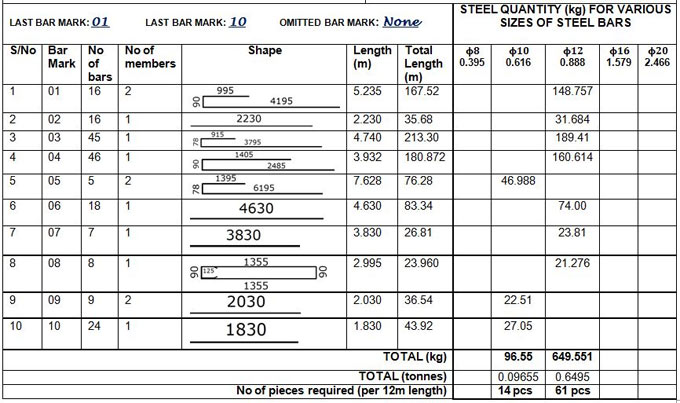# Bar Bending Schedule For Floor SlabsBar bending schedule is an important structural working document that rightly gives the disposition, bending shape, total length, and quantity of all the reinforcements that have been provided in a structural drawing.

It is often provided in a separate sheet (usually A4 paper) from the structural drawing.

The bar marks from structural detailing drawing are directly transferred to the bar bending schedule. We normally quantify reinforcements based on their total mass in tonnes or kilograms. For smaller projects, you can quantify based on the length needed.

Unit mass of rebars

The unit mass of the reinforcements is obtained from the density of steel. The density of steel provided for this purpose is 7850 kg/m3.

Suppose, there is a bar with 12mm dia;

The area is calculated by (πd2)/4 = (π × 122)/4 = 113.097mm2 = 0.0001131m2

Based on a unit length of the bar, it is established that the volume of a metre length of the bar is 0.0001131m3.

Density = Mass/Volume = 7850 kg/m3 = Mass/0.0001131

So, the unit mass of 12mm bar = 7850 × 0.0001131 = 0.888 kg/m

So, for any diameter of bar;

Basic weight = 0.00785 kg/mm2 per metre

Weight per metre = 0.006165 ϕ2 kg

Weight per mm2 at spacing s(mm) = 6.165ϕ2/s kg

Here;
ϕ denotes diameter of bar in millimetres
Bending Shapes
There exist some basic standard shapes with specific shape codes in the code of practice.
The length of reinforcement bars can be determined with the following relation;

Length of bar = Effective Length + Width of Support – Concrete cover (s) – Tolerances
The standard values of tolerances (deductions) are provided in the table below;

To get more details, go through the following link structville.com